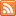Content Tagged “LO 4.4”Measures of Position

Published: Jul 20th, 2013

Percentiles Five-Number Summary Standardized Scores (Z-Scores) Measures of Position CO-4: Distinguish among different measurement scales, choose the appropriate descriptive and inferential statistical methods based on these distinctions, and interpret the results. […]

Describing Distributions

Published: Jun 28th, 2013

Features of Distributions of Quantitative Variables Shape (Symmetry/Skewness, Modality) Center Spread Outliers Let’s Summarize CO-4: Distinguish among different measurement scales, choose the appropriate descriptive and inferential statistical methods based on these distinctions, […]The “Normal” Shape

Published: Aug 21st, 2012

The Standard Deviation Rule Visual Methods of Assessing Normality Standardized Scores (Z-Scores) CO-4: Distinguish among different measurement scales, choose the appropriate descriptive and inferential statistical methods based on these distinctions, and […]Boxplots

Published: Aug 21st, 2012

The Five Number Summary The Boxplot Side-By-Side (Comparative) Boxplots Let’s Summarize CO-4: Distinguish among different measurement scales, choose the appropriate descriptive and inferential statistical methods based on these distinctions, and interpret […]Outliers

Published: Aug 21st, 2012

Using the IQR to Detect Outliers The 1.5(IQR) Criterion for Outliers The 3(IQR) Criterion for Outliers Understanding Outliers CO-4: Distinguish among different measurement scales, choose the appropriate descriptive and inferential statistical […]Published: Aug 21st, 2012

Introduction Range Inter-Quartile Range (IQR) Standard Deviation Properties of the Standard Deviation Choosing Numerical Measures Let’s Summarize CO-4: Distinguish among different measurement scales, choose the appropriate descriptive and inferential statistical methods […]Measures of Center

Published: Aug 21st, 2012

Introduction Mean Median Comparing the Mean and the Median Let’s Summarize CO-4: Distinguish among different measurement scales, choose the appropriate descriptive and inferential statistical methods based on these distinctions, and interpret […]Histograms & Stemplots

Published: Aug 19th, 2012

Histograms Stemplot (Stem and Leaf Plot) Let’s Summarize CO-4: Distinguish among different measurement scales, choose the appropriate descriptive and inferential statistical methods based on these distinctions, and interpret the results. LO […]

One Quantitative Variable

Published: Aug 19th, 2012

Distribution of One Quantitative Variable Graphs Numerical Measures CO-4: Distinguish among different measurement scales, choose the appropriate descriptive and inferential statistical methods based on these distinctions, and interpret the results. Video: One […]